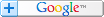# Microsoft Excel Training- From Beginner to Expert in 6 Hours/ EXCEL DASHBOARD REPORTS# Excel Average Formula/Function Without Including Zeros

## Omit Zeros from the Average Function/Formula

Back to Excel Formulas . Got any Excel Questions? Free Excel Help

Excel Average Without Zeros

Excel has a built in formula/function that makes averaging a range of cells easy. If we assume your numbers are in A1:A100 you would simply use the Average formula like shown below;

=AVERAGE(A1:A100)

There is however, one draw-back with this. That is, it includes cells that contain 0 (zeros). This can give you unexpected results. While the sum of values is not effected, the count of them is. Average is the sum of numbers divided by the count of numbers. So how do we omit zeros from our average?

SUMPRODUCT & SUM

=SUM(A1:A100)/(SUMPRODUCT((A1:A100<>0)*1))

This method is the most generic in that it ignores blank cells and will include negative numbers.

COUNTIF & SUM

By far the most efficient method is to use the SUM formula and COUNTIF formula as shown below;

=SUM(A1:A100)/COUNTIF(A1:A100,">0")

This method will not work should you have negative numbers unless you change ">0" to "<>0" The drawback is it will then count blank cells. Where as the one below wont.

=SUM(A1:A100) / (COUNT(A1:A100) - COUNTIF(A1:A100,0))

=SUMIF(A1:A100,">0")/COUNTIF(A1:A100,">0") Will also exclude negatives unless you use "<>0". However, it will then count blank cells.

If you do have negative numbers, and no blank cells, use;

=SUM(A1:A100)/COUNTIF(A1:A100,"<>0")

DAVERAGE

The other method is via the DAVERAGE function. This function is part of the Database functions and all are extremely useful when/if you need specify multiple criteria. The DAVERAGE , in the case of numbers being in A2:A100 (A1 should be a heading) we could use the DAVERAGE like below;

=DAVERAGE(\$A\$1:\$A\$100,1,\$B\$1:\$B\$2)

Where "1" represents the relative column position to average in the range A1:A100

B2 houses the expression >0

Array Average

This method is the least efficient. By creating an array formula we can use the Average formula as shown below to omit zeros;

=AVERAGE(IF(\$A\$1:\$A\$100,\$A\$1:\$A\$100))

=AVERAGE(IF(\$A\$1:\$A\$100 <>0,\$A\$1:\$A\$100))

As these are array formulas they MUST be entered via Ctrl+Shift+Enter.

Excel Dashboard Reports & Excel Dashboard Charts 50% Off Become an ExcelUser Affiliate & Earn Money

Special! Free Choice of Complete Excel Training Course OR Excel Add-ins Collection on all purchases totaling over \$64.00. ALL purchases totaling over \$150.00 gets you BOTH! Purchases MUST be made via this site. Send payment proof to [email protected] 31 days after purchase date.Search Tips FREE Excel Help